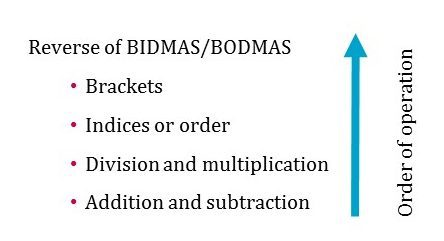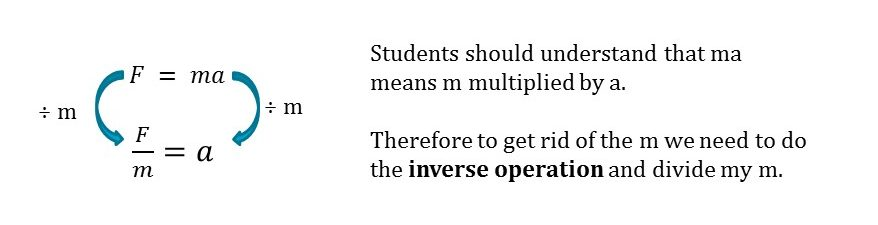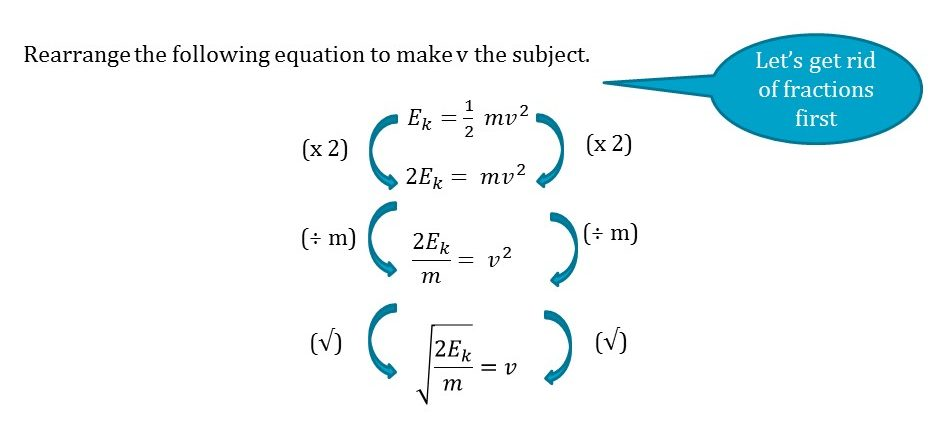# Rearranging equations in scienceIt’s fair to say I don’t find maths easy. That doesn’t mean I don’t appreciate the beauty of this subject because I do – I still remember discovering that π is the ratio of the circumference of any circle to the diameter, and not just a random number.

I just don’t fully understand it yet. Give me a calculation to solve and I will need to hold on tight, whilst suppressing some  tightly held misconceptions! For me:

• ¼ looks bigger than a ½  – it’s not, it’s smaller.
• ¼  should be 1:4 – it’s not, it’s 1:3 as there are only four parts.
• 3 + 4 x 2 should be 14 – it’s not, it’s 11

I’m not sure why I have to think so carefully when I do maths – I’ve done quite a lot over the years teaching A Level Chemistry and doing a PhD. It’s a similar feeling to when I speak French. I think I’ve learnt the rules without actually understanding the logic that sits beneath them – it’s time to change all of that with some help from Amie Barr!

#### Rearranging equations in science

Let’s start with what an equation is. An equation is a mathematical statement that indicates the equality of the expressions to the left and right of the equals sign (Language of Mathematics in Science, p.87). In other words, an equation is a statement about the equality between things:

number of children in the class = number of boys + number of girls

F = ma

y = x + 2

A formula is an equation that shows the relationship between physical quantities. Every formula is an equation but not every equation is a formula. For example, F = ma is a formula and an equation because it shows the relationship between the physical quantities force, mass and acceleration. y = x + 2 is an equation but not a formula because the variables are abstract. The word variable here refers to any part of an equation, commonly an alphabetic character, that can take on any value e.g. x > 5 or 2 x = 10 or F=ma.

#### Rearranging equations: the concept of equality

Before you teach students to rearrange an equation containing letters, start with some simpler examples using numbers. This helps students appreciate the important idea of maintaining equality when changes are made to an equation.

5 + 4 = 9 [this is true]

5 + 4 + 3 = 9 + 3 [this is also true]

5 + 4 – 2 = 9 – 2 [and so is this]

5 + 4 – 2 = 9 – 3 [this is not true – ERROR –  the rule of equality has been broken]

#### Rearranging equations: orders of operation

Once students understand that equality must be maintained in an equation, you can then consider the order in which you carry out operations (addition/subtraction/multiplication and division) when manipulating equations.For example,   3 + 4 x 2 is 11 and not 14.

This is because multiplication should be done before addition – see the BIDMAS graphic to help.

3 + 4 x 2

3 + 8

11

Where expressions involve either only addition or subtraction, or division and multiplication, the operations are carried out from left to right.

3 x 8 ÷ 2 ÷ 2

24 ÷ 2 ÷ 2

12 ÷ 2

6

#### Rearranging equations: one step at a time

Let’s look now at how we can teach students to show their working (thinking!) when rearranging an equation to change the subject. Make sure students first understand the concepts of equality and order of operations. Here is a potential method of rearrangement that you could use.Step 1: write the equation

Step 2: carry out inverse operation(s). One per step.

Students may ask why the m on the right-hand side disappeared. It is worth spending time discussing what has actually happened here using the below examples.

4 ÷4 = 1

7 ÷7 = 1

h ÷ h = 1

3a ÷ 3 = 1a

ma ÷ m = a

Let’s look at a more complicated example involving kinetic energy. What’s important here is that we only perform one operation at each stage.#### Equations are useful!

Finally, it’s important that students see equations as helpful ‘tools’ and not just things that need to be learnt. For example, equations can be very helpful in allowing us to understand and determine units. You can download an activity to help students see where units come from here (PDF).  Let’s look at an example below.

Imagine you are asked for the unit for speed but can’t remember it. You do though know the equation:

speed = distance ÷ time

this is the same as writing speed = distance/time

You can now work out the units of speed by substituting into the equation the units for distance and time.

speed = m/s

so unit of speed = m/s

Let’s try a harder one. What is the unit of concentration?

concentration = moles ÷ volume

this is the same as writing concentration = moles/volume

so unit of concentration  = mol/dm³

It’s worth noting that at A Level the same unit of concentration is written as mol dm3

How is this possible?!  Let’s look at another example.

1 ÷ 6 = 6-1

1 ÷ x = x-1

mol/dm³ = mol ÷ dm³ = mol dm3

You could also work in the other direction. Let’s say that you know that the unit for torque (moment) is Newton metres (Nm). This immediately allows us to see how torque is calculated i.e. torque =  force x distance. In a similar way you can determine the equation for pressure from its units (N/m2). pressure = force ÷ area

#### Summary

As science teachers we have a duty to help students to understand the concepts they are using  –   even if we ourselves need to do some brushing up first! Where possible, work with your maths department to agree a common way of teaching the same concept and run some training together.

Do:

• Read Chapter 9 from the Language of Mathematics in Science
• link rearranging to solving equations. We really want students to see these two skills as the same thing.
• make sure students appreciate the concept of equality first  – using simple equations with numbers can help.
• when using a formula, substitute in the values first, simplify everything you can and then rearrange. Students find this easier than first rearranging.
• show clearly what is being done to get from one step to the next. Modelling is key here. Ask students to use the teacher whiteboard to write down their working and show their thinking.

Don’t:

• talk about the symbol changing as it crosses the magic equals!
• use formula triangles! They don’t develop understanding of rearrangement or units and are limited to simple. equations. They definitely won’t set students up for A Level and beyond.
• write the working in the equations i.e.

y = mx + c

y – c = mx + c – c

Have fun with equations in science. It’s a whole new world to explore with incredible explanatory power.